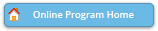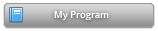#### Abstract Details

 Activity Number: 79 - Functional Data Analysis: Methods and Applications Type: Contributed Date/Time: Sunday, July 28, 2019 : 4:00 PM to 5:50 PM Sponsor: Section on Statistical Learning and Data Science Abstract #305366 Presentation Title: Historical and Restricted Function-On-Function Regression Models Author(s): Ruiyan Luo* and Xin Qi Companies: Georgia State University and Georgia State University Keywords: functional data; function-on-function regression; historical model Abstract: In historical function-on-function regression model, it is assumed that the value of the response curve at a time point ($y(t)$) only depends on the values of the predictor curves before this time point ($x(s)$ for $s< t$). Equivalently, the model can be expressed in terms of the integral of the multiplication of coefficient kernel $\beta(s,t)$ and the predictor curve $x(s)$ in a triangular subregion $s\le t$. We consider more general and flexible models, where the integral $\int x(s)\beta(s,t) ds$ of the multiplication of coefficient kernel and the predictor curve can be taken in any subregion. Given a subregion, we propose a decomposition of the coefficient kernel and algorithms to jointly estimate the decomposition. When the subregion is unknown, we propose a method to simultaneously determine the subregion and estimate the coefficient kernel.

Authors who are presenting talks have a * after their name.